## Contiguous Memory Allocation-

Before you go through this article, make sure that you have gone through the previous articles on Static Partitioning and Dynamic Partitioning.

There are two popular techniques used for contiguous memory allocation-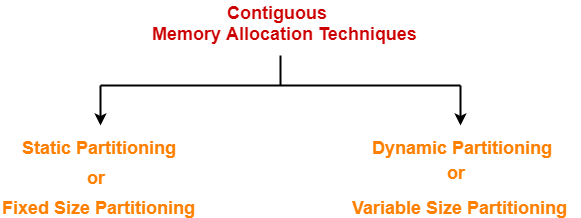In this article, we will discuss practice problems based on Contiguous Memory Allocation.

## Problem-01:

Consider six memory partitions of size 200 KB, 400 KB, 600 KB, 500 KB, 300 KB and 250 KB. These partitions need to be allocated to four processes of sizes 357 KB, 210 KB, 468 KB and 491 KB in that order.

Perform the allocation of processes using-

1. First Fit Algorithm
2. Best Fit Algorithm
3. Worst Fit Algorithm

## Solution-

According to question,

The main memory has been divided into fixed size partitions as-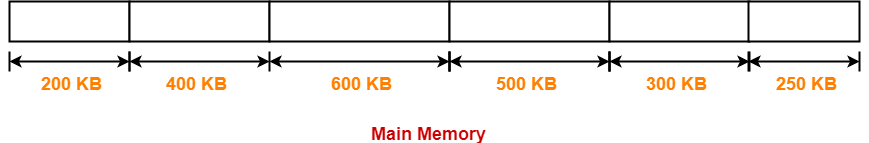Let us say the given processes are-

• Process P1 = 357 KB
• Process P2 = 210 KB
• Process P3 = 468 KB
• Process P4 = 491 KB

## Allocation Using First Fit Algorithm-

In First Fit Algorithm,

• Algorithm starts scanning the partitions serially.
• When a partition big enough to store the process is found, it allocates that partition to the process.

The allocation of partitions to the given processes is shown below-

### Step-01: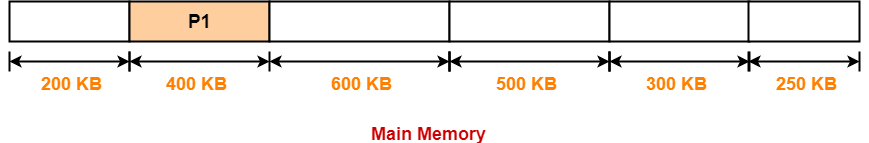### Step-02: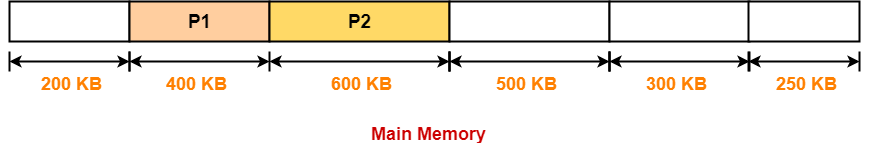### Step-03: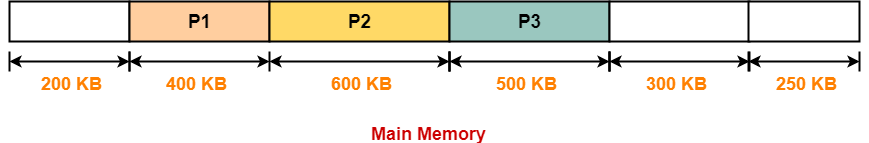### Step-04:

• Process P4 can not be allocated the memory.
• This is because no partition of size greater than or equal to the size of process P4 is available.

## Allocation Using Best Fit Algorithm-

In Best Fit Algorithm,

• Algorithm first scans all the partitions.
• It then allocates the partition of smallest size that can store the process.

The allocation of partitions to the given processes is shown below-

### Step-01:### Step-02: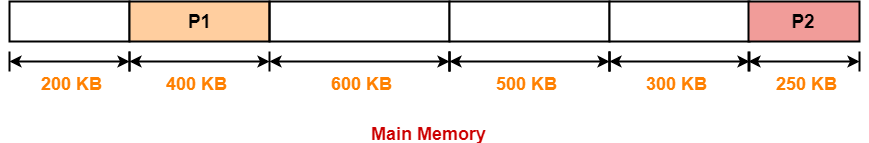### Step-03: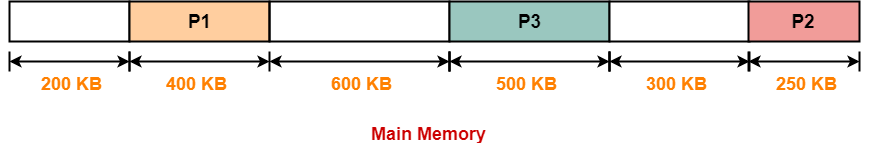### Step-04: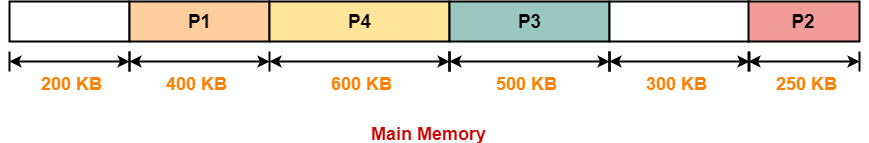## Allocation Using Worst Fit Algorithm-

In Worst Fit Algorithm,

• Algorithm first scans all the partitions.
• It then allocates the partition of largest size to the process.

The allocation of partitions to the given processes is shown below-

### Step-01: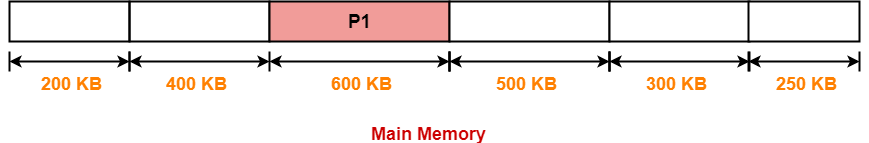### Step-02: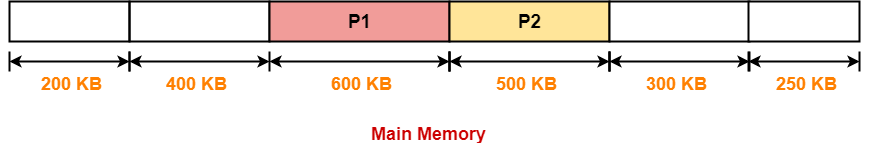### Step-03:

• Process P3 and Process P4 can not be allocated the memory.
• This is because no partition of size greater than or equal to the size of process P3 and process P4 is available.

## Problem-02:

Consider the following heap (figure) in which blank regions are not in use and hatched regions are in use-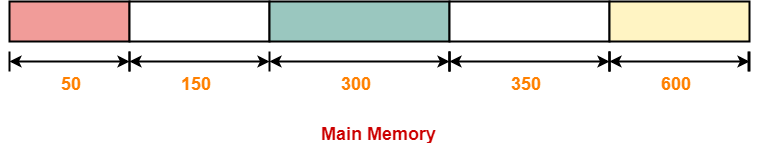The sequence of requests for blocks of size 300, 25, 125, 50 can be satisfied if we use-

1. Either first fit or best fit policy (any one)
2. First fit but not best fit policy
3. Best fit but not first fit policy
4. None of the above

## Solution-

The allocation follows variable size partitioning scheme.

Let us say the given processes are-

• Process P1 = 300 units
• Process P2 = 25 units
• Process P3 = 125 units
• Process P4 = 50 units

## Allocation Using First Fit Algorithm-

The allocation of partitions to the given processes is shown below-

### Step-01: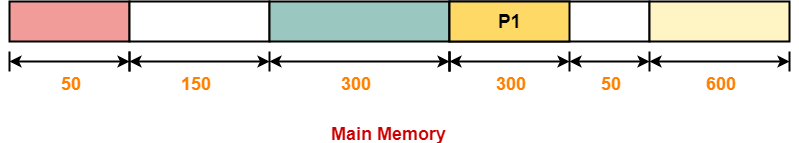### Step-02: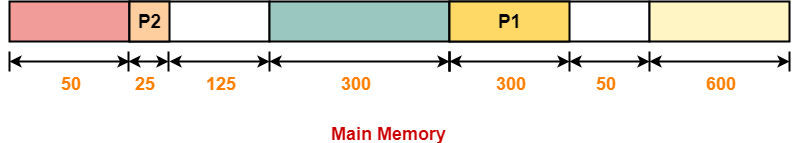### Step-03: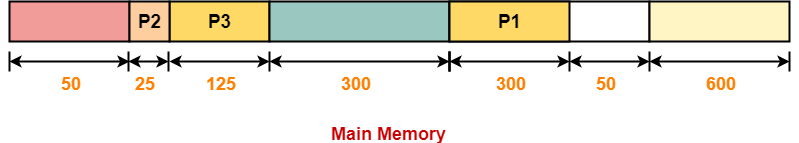### Step-04: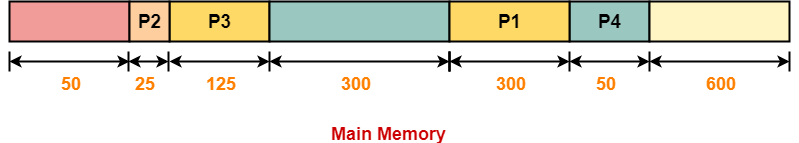## Allocation Using Best Fit Algorithm-

The allocation of partitions to the given processes is shown below-

### Step-01:### Step-02: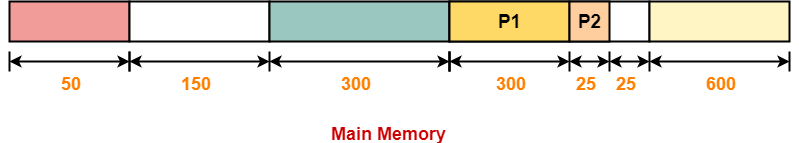### Step-03: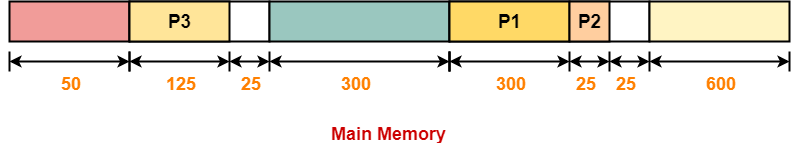### Step-04:

• Process P4 can not be allocated the memory.
• This is because no partition of size greater than or equal to the size of process P4 is available.

Thus,

• Only first fit allocation policy succeeds in allocating memory to all the processes.
• Option (B) is correct.

Next Article-Introduction to Paging

Get more notes and other study material of Operating System.

Watch video lectures by visiting our YouTube channel LearnVidFun.

## Contiguous Memory Allocation-

Before you go through this article, make sure that you have gone through the previous article on Contiguous Memory Allocation.

We have discussed-

• In contiguous memory allocation, a process can be stored only in a contiguous fashion.
• There are two popular techniques used for contiguous memory allocation-## Dynamic Partitioning-

• Dynamic partitioning is a variable size partitioning scheme.
• It performs the allocation dynamically.
• When a process arrives, a partition of size equal to the size of process is created.
• Then, that partition is allocated to the process.

## Partition Allocation Algorithms-

• The processes arrive and leave the main memory.
• As a result, holes of different size are created in the main memory.
• These holes are allocated to the processes that arrive in future.

 Partition allocation algorithms are used to decide which hole should be allocated to the arrived process.

Popular partition allocation algorithms are-1. First Fit Algorithm
2. Best Fit Algorithm
3. Worst Fit Algorithm

We have already discussed these algorithms in our previous article.

## Important Points-

### Point-01:

For dynamic partitioning,

• Worst Fit Algorithm works best.
• This is because space left after allocation inside the partition is of large size.
• There is a high probability that this space might suit the requirement of arriving processes.

### Point-02:

For dynamic partitioning,

• Best Fit Algorithm works worst.
• This is because space left after allocation inside the partition is of very small size.
• There is a low probability that this space might suit the requirement of arriving processes.

The following diagram illustrates the steps of translating logical address into physical address-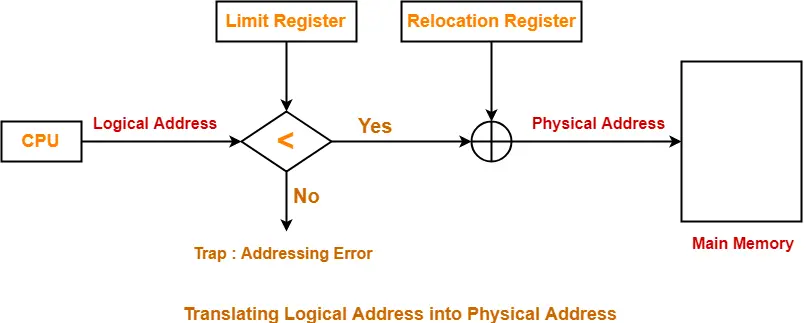The steps are same as we have discussed in our previous article.

The advantages of dynamic partitioning are-

• It does not suffer from internal fragmentation.
• Degree of multiprogramming is dynamic.
• There is no limitation on the size of processes.

The disadvantages of dynamic partitioning are-

• It suffers from external fragmentation.
• Allocation and deallocation of memory is complex.

To gain better understanding about Contiguous Memory Allocation,

Watch this Video Lecture

Next Article-Practice Problems On Contiguous Memory Allocation

Get more notes and other study material of Operating System.

Watch video lectures by visiting our YouTube channel LearnVidFun.

## Contiguous Memory Allocation-

• Contiguous memory allocation is a memory allocation technique.
• It allows to store the process only in a contiguous fashion.
• Thus, entire process has to be stored as a single entity at one place inside the memory.

## Techniques-

There are two popular techniques used for contiguous memory allocation-1. Static Partitioning
2. Dynamic Partitioning

## Static Partitioning-

• Static partitioning is a fixed size partitioning scheme.
• In this technique, main memory is pre-divided into fixed size partitions.
• The size of each partition is fixed and can not be changed.
• Each partition is allowed to store only one process.

## Example-

Under fixed size partitioning scheme, a memory of size 10 KB may be divided into fixed size partitions as-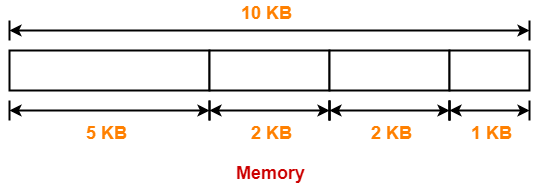• These partitions are allocated to the processes as they arrive.
• The partition allocated to the arrived process depends on the algorithm followed.

## Algorithms for Partition Allocation-

Popular algorithms used for allocating the partitions to the arriving processes are-1. First Fit Algorithm
2. Best Fit Algorithm
3. Worst Fit Algorithm

## 1. First Fit Algorithm-

• This algorithm starts scanning the partitions serially from the starting.
• When an empty partition that is big enough to store the process is found, it is allocated to the process.
• Obviously, the partition size has to be greater than or at least equal to the process size.

## 2. Best Fit Algorithm-

• This algorithm first scans all the empty partitions.
• It then allocates the smallest size partition to the process.

## 3. Worst Fit Algorithm-

• This algorithm first scans all the empty partitions.
• It then allocates the largest size partition to the process.

## Important Points-

### Point-01:

For static partitioning,

• Best Fit Algorithm works best.
• This is because space left after the allocation inside the partition is of very small size.
• Thus, internal fragmentation is least.

### Point-02:

For static partitioning,

• Worst Fit Algorithm works worst.
• This is because space left after the allocation inside the partition is of very large size.
• Thus, internal fragmentation is maximum.

### Internal Fragmentation

• It occurs when the space is left inside the partition after allocating the partition to a process.
• This space is called as internally fragmented space.
• This space can not be allocated to any other process.
• This is because only static partitioning allows to store only one process in each partition.
• Internal Fragmentation occurs only in static partitioning.

### External Fragmentation

• It occurs when the total amount of empty space required to store the process is available in the main memory.
• But because the space is not contiguous, so the process can not be stored.

• CPU always generates a logical address.
• A physical address is needed to access the main memory.

## Step-01:

• The translation scheme uses two registers that are under the control of operating system.
• During context switching, the values corresponding to the process being loaded are set in the registers.

These two registers are-

• Relocation Register
• Limit Register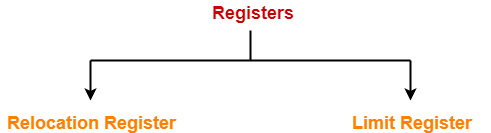• Relocation Register stores the base address or starting address of the process in the main memory.
• Limit Register stores the size or length of the process.

## Step-02:

• CPU generates a logical address containing the address of the instruction that it wants to read.

## Step-03:

• The logical address generated by the CPU is compared with the limit of the process.
• Now, two cases are possible-

### Case-01: Generated Address >= Limit

• If address is found to be greater than or equal to the limit, a trap is generated.
• This helps to prevent unauthorized access.

### Case-02: Generated Address < Limit

• The address must always lie in the range [0, limit-1].
• If address is found to be smaller than the limit, then the request is treated as a valid request.
• The result obtained after addition is the address of the memory location storing the required word.

## Diagram-

The following diagram illustrates the above steps of translating logical address into physical address-The advantages of static partitioning are-

• It is simple and easy to implement.
• It supports multiprogramming since multiple processes can be stored inside the main memory.
• Only one memory access is required which reduces the access time.

The disadvantages of static partitioning are-

• It suffers from both internal fragmentation and external fragmentation.
• It utilizes memory inefficiently.
• The degree of multiprogramming is limited equal to number of partitions.
• There is a limitation on the size of process since processes with size greater than the size of largest partition can’t be stored and executed.

To gain better understanding about Contiguous Memory Allocation,

Watch this Video Lecture

Next Article-Dynamic Partitioning

Get more notes and other study material of Operating System.

Watch video lectures by visiting our YouTube channel LearnVidFun.

## Paging in OS-

Before you go through this article, make sure that you have gone through the previous article on Paging in OS.

We have discussed-

• Paging is a non-contiguous memory allocation technique.
• Page Table keeps track of the frames storing the pages of the process.

In paging scheme, there are mainly two overheads-

### 1. Overhead of Page Tables-

• Paging requires each process to maintain a page table.
• So, there is an overhead of maintaining a page table for each process.

### 2. Overhead of Wasting Pages-

• There is an overhead of wasting last page of each process if it is not completely filled.
• On an average, half page is wasted for each process.

Thus,

= Size of its page table + (Page size / 2)

## Optimal Page Size-

Optimal page size is the page size that minimizes the total overhead.

It is given as-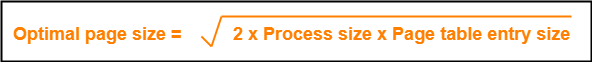## Proof-

Total overhead due to one process

= Size of its page table + (Page size / 2)

= Number of entries x Page table entry size + (Page size / 2)

= Number of pages the process is divided x Page table entry size + (Page size / 2)

= (Process size / Page size) x Page table entry size + (Page size / 2)

Now,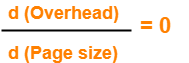Keeping process size and page table entry size as constant, differentiating overhead with respect to page size, we get-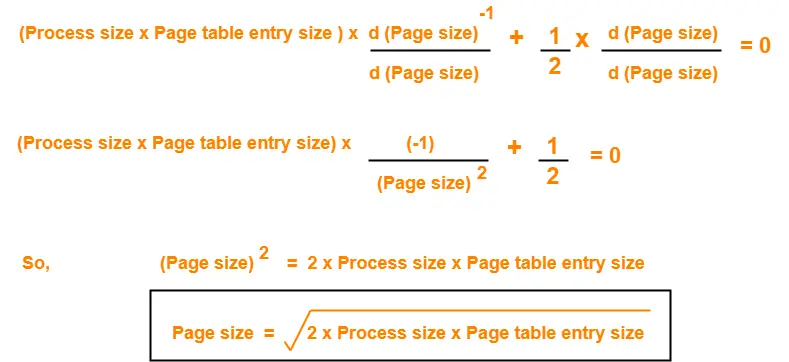## Problem-01:

In a paging scheme, virtual address space is 4 KB and page table entry size is 8 bytes. What should be the optimal page size?

## Solution-

Given-

• Virtual address space = Process size = 4 KB
• Page table entry size = 8 bytes

We know-

Optimal page size

= (2 x Process size x Page table entry size)1/2

= (2 x 4 KB x 8 bytes)1/2

= (216 bytes x bytes)1/2

= 28 bytes

= 256 bytes

Thus, Optimal page size = 256 bytes.

## Problem-02:

In a paging scheme, virtual address space is 16 MB and page table entry size is 2 bytes. What should be the optimal page size?

## Solution-

Given-

• Virtual address space = Process size = 16 MB
• Page table entry size = 2 bytes

We know-

Optimal page size

= (2 x Process size x Page table entry size)1/2

= (2 x 16 MB x 2 bytes)1/2

= (226 bytes x bytes)1/2

= 213 bytes

= 8 KB

Thus, Optimal page size = 8 KB.

## Problem-03:

In a paging scheme, virtual address space is 256 GB and page table entry size is 32 bytes. What should be the optimal page size?

## Solution-

Given-

• Virtual address space = Process size = 256 GB
• Page table entry size = 32 bytes

We know-

Optimal page size

= (2 x Process size x Page table entry size)1/2

= (2 x 256 GB x 32 bytes)1/2

= (244 bytes x bytes)1/2

= 222 bytes

= 4 MB

Thus, Optimal page size = 4 MB.

Next Article-Practice Problems On Paging in OS

Get more notes and other study material of Operating System.

Watch video lectures by visiting our YouTube channel LearnVidFun.

## Segmented Paging-

Before you go through this article, make sure that you have gone through the previous article on Segmented Paging.

We have discussed-

• Segmented Paging is a scheme that implements the combination of Segmentation and Paging.
• First, segmentation divides the process into segments.
• Then, paging divides each segment into pages.

In this article, we will discuss practice problems based on segmented paging.

## Problem-01:

A certain computer system has the segmented paging architecture for virtual memory. The memory is byte addressable. Both virtual and physical address spaces contain 216 bytes each. The virtual address space is divided into 8 non-overlapping equal size segments. The memory management unit (MMU) has a hardware segment table, each entry of which contains the physical address of the page table for the segment. Page tables are stored in the main memory and consists of 2 byte page table entries. What is the minimum page size in bytes so that the page table for a segment requires at most one page to store it?

## Solution-

Given-

• Virtual Address Space = Process size = 216 bytes
• Physical Address Space = Main Memory size = 216 bytes
• Process is divided into 8 equal size segments
• Page table entry size = 2 bytes

Let page size = n bytes.

Now, since page table has to be stored into a single page, so we must have-

Size of page table <= Page size

### Size of Each Segment-

Size of each segment

= Process size / Number of segments

= 216 bytes / 8

= 216 bytes / 23

= 213 bytes

= 8 KB

### Number of Pages Of Each Segment-

Number of pages each segment is divided

= Size of segment / Page size

= 8 KB / n bytes

= (8K / n) pages

### Size of Each Page Table-

Size of each page table

= Number of entries in page table x Page table entry size

= Number of pages the segment is divided x 2 bytes

= (8K / n) x 2 bytes

= (16K / n) bytes

### Page Size-

Substituting values in the above condition, we get-

(16K / n) bytes <= n bytes

(16K / n) <= n

n2 >= 16K

n2 >= 214

n >= 27

Thus, minimum page size possible = 27 bytes = 128 bytes.

## Problem-02:

Considering problem-01, give the division of virtual address.

## Solution-

### Number of Bits Required For Segment Number-

Number of segments the process is divided

= 8

= 23

Thus, Number of bits required to identify a particular segment in segment table = 3 bits.

### Number of Bits Required For Page Number-

Number of pages a segment is divided

= Segment size / Page size

= 8KB / 128 bytes

= 213 bytes / 27 bytes

= 26 pages

Thus, Number of bits required to identify a particular page in table = 6 bits.

### Number of Bits Required For Page Offset-

Page size

= 128 bytes

= 27 bytes

Thus, Number of bits required for page offset = 7 bits.

Thus, virtual address is divided as-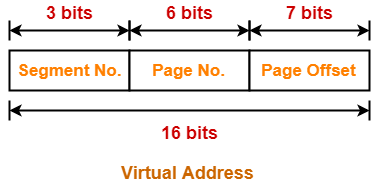## Problem-03:

A certain computer system has the segmented paging architecture for virtual memory. The memory is byte addressable. Both virtual and physical address spaces contain 216 bytes each. The virtual address space is divided into 8 non-overlapping equal size segments. The memory management unit (MMU) has a hardware segment table, each entry of which contains the physical address of the page table for the segment. Page tables are stored in the main memory and consists of 2 byte page table entries.. Assume that each page table entry contains (besides other information) 1 valid bit, 3 bits for page protection and 1 dirty bit. How many bits are available in page table entry for storing the aging information for the page? Assume that page size is 512 bytes.

## Solution-

Given-

• Virtual Address Space = Process size = 216 bytes
• Physical Address Space = Main Memory size = 216 bytes
• Process is divided into 8 equal size segments
• Page table entry size = 2 bytes = 16 bits
• Page table entry besides other information contains 1 valid bit, 3 protection bits, 1 dirty bit
• Page size = 512 bytes

### Number of Frames in Main Memory-

Number of frames in main memory

= Size of main memory / Page size

= 216 bytes / 512 bytes

= 216 bytes / 29 bytes

= 27 frames

Thus, Number of bits required for frame identification in page table entry = 7 bits

### Number Of Bits Available For Storing Aging Information-

Number of bits available for storing aging information

= Number of bits in page table entry – ( Number of bits required for frame identification + 1 valid bit + 3 protection bits + 1 dirty bit)

= 16 bits – (7 + 1 + 3 + 1) bits

= 16 bits – 12 bits

= 4 bits

Next Article-Disk Scheduling Algorithms

Get more notes and other study material of Operating System.

Watch video lectures by visiting our YouTube channel LearnVidFun.